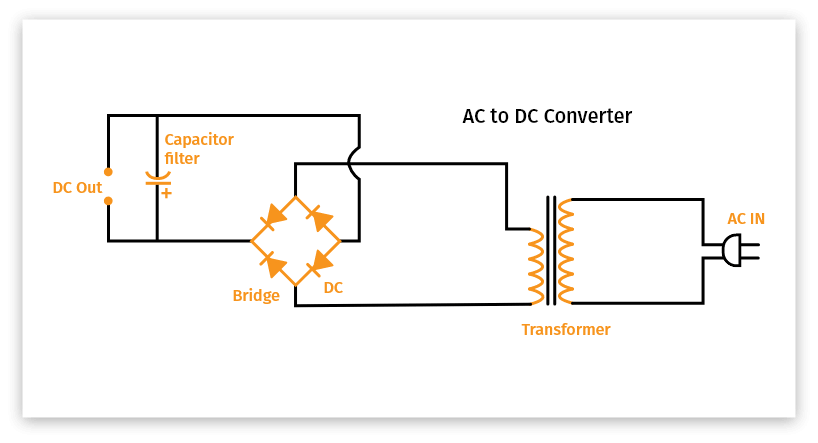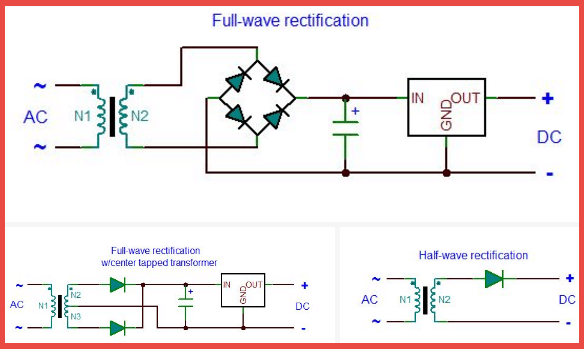# AC to DC Calculator

To convert ac voltage to dc voltage enter value in given field by using our ac to dc converter calculator.## Formula

DC to AC conversion formula
AC = DC / 0.636
AC to DC conversion formula
DC = AC * 0.636

Where,
AC - Alternating Current
DC - Direct Current
0.636 - Constant

This Calculator is combination of ac to dc calculator and dc to ac calculator. AC and DC are electric supply current adaptors. AC refers to Alternating Current and DC refers to Direct Current.

An alternating current (AC) refers to an electrical current whose magnitude and direction vary cyclically, as opposed to direct current (DC). The direction of Direct Current (DC) always remains constant.

Direct current (DC) is used to charge batteries, and also used in nearly all electronic systems as the power supply. Electric power that is being distributed all over is nearly all Alternating Current (AC) today.The advanced online AC to DC Converter is used to calculate and convert the electric currents from Alternating Current (AC) to Direct Current (DC) by applying the formula.

Example:
how to convert ac to dc using this formula
Convert the given value of Direct Current (DC) to Alternating Current (AC).
DC Current = 15

Solution:
Apply Formula:
AC = DC / 0.636
AC = 15/0.636
AC = 23.584 volts
AC Current = 23.584 volt

Structure Diagram of AC to DC shown below.1. MIT School of Engineering | » What’s the difference between AC and DC?. Mit Engineering.
2. AC vs DC (Alternating Current vs Direct Current) - Difference and Comparison | Diffen.com
3. Alternating Current (AC) vs. Direct Current (DC) - Learn.sparkfun.com.